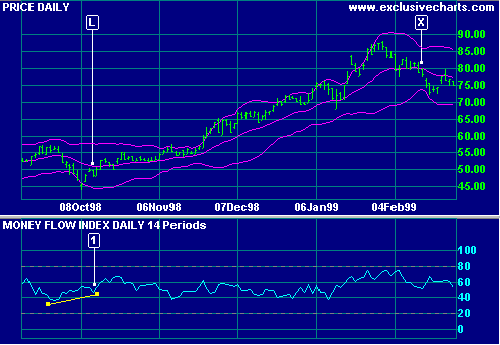# Money Flow Index

The Money Flow Index is a volume-weighted version of the Relative Strength Index, used to warn of trend weakness and likely reversal points. The indicator compares the value traded on up-days to value traded on down-days.

### Ranging Market

Ranging markets can be identified by Money Flow Index fluctuating close to the 50 level.

### Trending Market

Market tops are likely when a medium term Money Flow Index is above 80.

Market bottoms are likely when a medium term Money Flow Index is below 20.

Only trade with the trend and exit using a trend indicator.

#### Example

Microsoft Corporation plotted with    Bollinger bands at 2.0 standard deviations around a 20 day exponential moving average and    20 day Money Flow Index.Mouse over chart captions to display trading signals.

Exit using the moving average - this example uses MA direction as a filter. Compare this to the results if a single close or 2 closes are used as a filter.

1. Go long [L] on a bullish divergence. Exit when the moving average turns down at [X].
##### Setup

The default Money Flow Index window is 14 days. Overbought/oversold are set at 80% and 20% levels. To alter the default settings - Edit Indicator Settings.

See Indicator Panel for directions on how to set up an indicator.

## Formula

1. Calculate Typical Price for each period:
(High + Low + Close) / 3

2. Calculate Money Flow for each period:
Typical Price * Volume

3. Decide on the time frame over which to calculate the index. This should be based on the cycle  that you are trading.

4. Calculate Positive Money Flow:
Add Money Flow for each period (in the time frame) that Typical Price moves up.

5. Calculate Negative Money Flow:
Add Money Flow for each period (in the time frame) that Typical Price moves down.

6. Calculate the Money Ratio:
Positive Money Flow / Negative Money Flow

7. Calculate the Money Flow Index:
100 - 100 / (1+ Money Ratio)